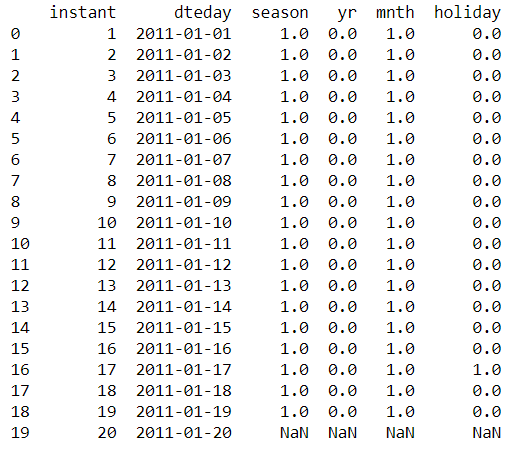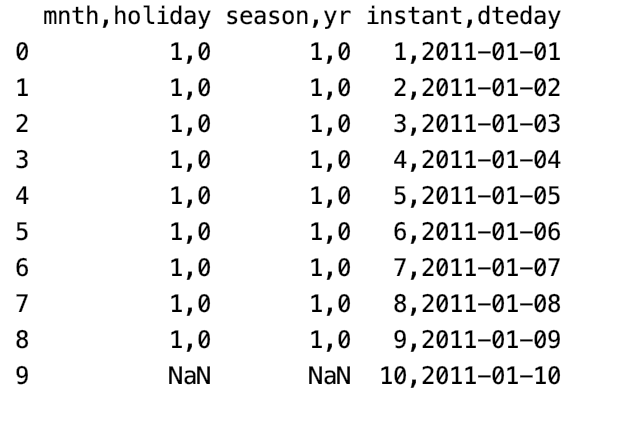# How to read multiple data files into Pandas?

• Last Updated : 23 Aug, 2021

In this article, we are going to see how to read multiple data files into pandas, data files are of multiple types, here are a few ways to read multiple files by using the pandas package in python.

Attention geek! Strengthen your foundations with the Python Programming Foundation Course and learn the basics.

To begin with, your interview preparations Enhance your Data Structures concepts with the Python DS Course. And to begin with your Machine Learning Journey, join the Machine Learning - Basic Level Course

## Method 1: Reading CSV files

If our data files are in CSV format then the read_csv() method must be used. read_csv takes a file path as an argument. it reads the content of the CSV. To read multiple CSV files we can just use a simple for loop and iterate over all the files.

Example: Reading Multiple CSV files using Pandas

In this example we make a list of our data files or file path and then iterate through the file paths using a for loop, a for loop is used to iterate through iterables like list, tuples, strings, etc. And then create a data frame using pd.DataFrame(), concatenate each dataframe into a main dataframe using pd.concat(), then convert the final main dataframe into a CSV file using to_csv() method which takes the name of the new CSV file we want to create as an argument.

## Python3

 `# importing pandas``import` `pandas as pd`` ` `file_list``=``[``'a.csv'``,``'b.csv'``,``'c.csv'``]`` ` `main_dataframe ``=` `pd.DataFrame(pd.read_csv(file_list[``0``]))`` ` `for` `i ``in` `range``(``1``,``len``(file_list)):``    ``data ``=` `pd.read_csv(file_list[i])``    ``df ``=` `pd.DataFrame(data)``    ``main_dataframe ``=` `pd.concat([main_dataframe,df],axis``=``1``)``print``(main_dataframe)`

Output:## Method 2: Using the glob package

The glob module in python is used to retrieve files or pathnames matching a specified pattern.

This program is similar to the above program but the only difference is instead of keeping track of file names using a list we use the glob package to retrieve files matching a specified pattern.

Example: Reading multiple CSV files using Pandas and glob.

## Python3

 `# importing packages``import` `pandas as pd``import` `glob`` ` `folder_path ``=` `'Path_of_file/csv_files'``file_list ``=` `glob.glob(folder_path ``+` `"/*.csv"``)``main_dataframe ``=` `pd.DataFrame(pd.read_csv(file_list[``0``]))``for` `i ``in` `range``(``1``,``len``(file_list)):``    ``data ``=` `pd.read_csv(file_list[i])``    ``df ``=` `pd.DataFrame(data)``    ``main_dataframe ``=` `pd.concat([main_dataframe,df],axis``=``1``)``print``(main_dataframe)`

Output:## Method 3: Reading text files using Pandas:

Example: Reading text file using pandas and glob.

Using glob package to retrieve files or pathnames and then iterate through the file paths using a for loop. Create a data frame of the contents of each file after reading it using pd.read_table() method which takes the file path as an argument. Concatenate each dataframe into a main dataframe using pd.concat(), then convert the final main dataframe into a CSV file using to_csv() method which takes the name of the new CSV file we want to create as an argument.

## Python3

 `# importing packages``import` `pandas as pd``import` `glob`` ` `folder_path ``=` `'Path_/files'``file_list ``=` `glob.glob(folder_path ``+` `"/*.txt"``)``main_dataframe ``=` `pd.DataFrame(pd.read_table(file_list[``0``]))`` ` `for` `i ``in` `range``(``1``,``len``(file_list)):``    ``data ``=` `pd.read_table(file_list[i])``    ``df ``=` `pd.DataFrame(data)``    ``main_dataframe ``=` `pd.concat([main_dataframe, df], axis ``=` `1``)`` ` `print``(main_dataframe)`` ` `# creating a new csv file with``# the dataframe we created``main_dataframe.to_csv(``'new_csv1.csv'``)`

Output:My Personal Notes arrow_drop_up